## WitCH 36: Sub Standard

This WitCH is a companion to our previous, MitPY post, and is a little different from most of our WitCHes. Typically in a WitCH the sin is unarguable, and it is only the egregiousness of the sin that is up for debate. In this case, however, there is room for disagreement, along with some blatant sinning. It comes, predictably, from Cambridge’s Specialist Mathematics 3 & 4 (2020).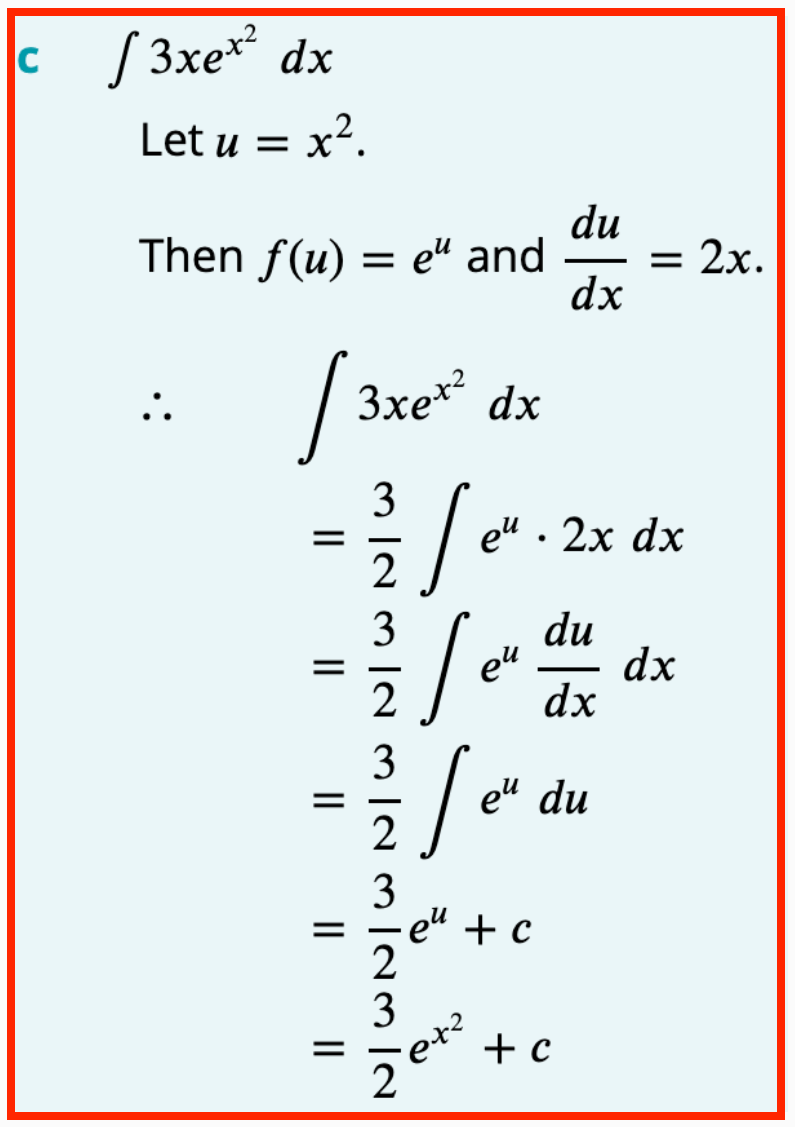## WitCH 15: Principled Objection

OK, playtime is over. This one, like the still unresolved WitCH 8, will take some work. It comes from Cambridge’s Mathematical Methods 3 & 4 (2019). It is the introduction to “When is a function differentiable?”, the final section of the chapter “Differentiation”.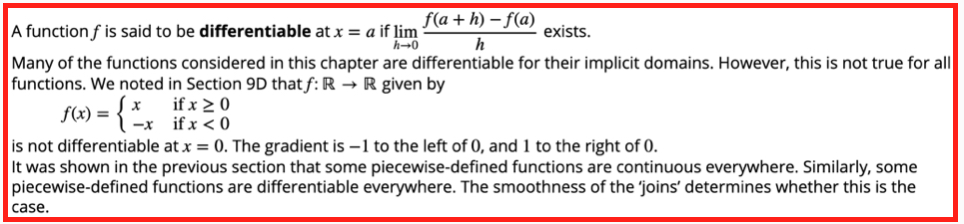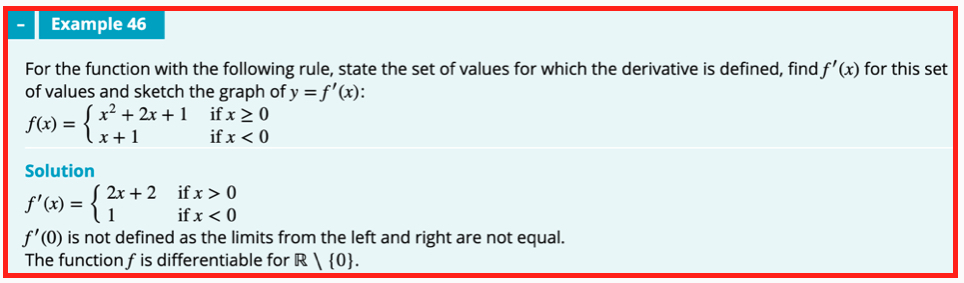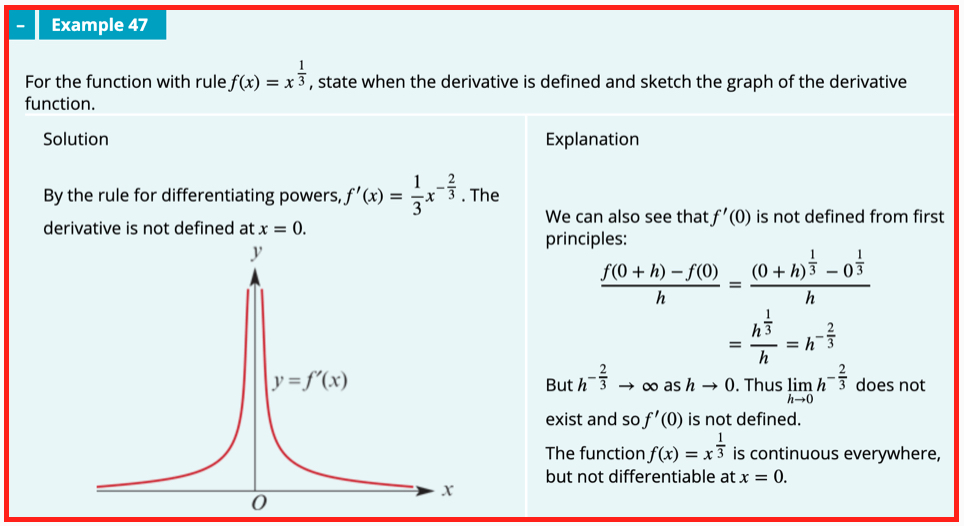## Update (12/08/19)

We wrote about this nonsense seven long years ago, and we’ll presumably be writing about it seven years from now. Nonetheless, here we go.

The first thing to say is that the text is wrong. To the extent that there is a discernible method, that method is fundamentally invalid. Indeed, this is just about the first nonsense whacked out of first year uni students.

The second thing to say is that the text is worse than wrong. The discussion is clouded in gratuitous mystery, with the long-delayed discussion of “differentiability” presented as some deep concept, rather than simply as a grammatical form. If a function has a derivative then it is differentiable. That’s it.

Now to the details.

The text’s “first principles” definition of differentiability is correct and then, immediately, things go off the rails. Why is the function f(x) = |x| (which is written in idiotic Methods style) not differentiable at 0? The wording is muddy, but example 46 makes clear the argument: f’(x) = -1 for x < 0 and f’(x) = 1 for x > 0, and these derivatives don’t match. This argument is unjustified, fundamentally distinct from first principles, and it can easily lead to error. (Amusingly, the text’s earlier, “informal” discussion of f(x) = |x| is exactly what is required.)

The limit definition of the derivative f’(a) requires looking precisely at a, at the gradient [f(a+h) – f(a)]/h as h → 0. Instead, the text, with varying degrees of explicitness and correctness, considers the limit of f’(x) near a, as x → a. This second limit is fundamentally, conceptually different and it is not guaranteed to be equal.

The standard example to illustrate the issue is the function f(x) = x2sin(1/x) (for x≠ 0 and with f(0) = 0). It is easy to to check that f’(x) oscillates wildly near 0, and thus f’(x) has no limit as x → 0. Nonetheless, a first principles argument shows that f’(0) = 0.

It is true that if a function f is continuous at a, and if f’(x) has a limit L as x → a, then also f’(a) = L. With some work, this non-obvious truth (requiring the mean value theorem) can be used to clarify and to repair the text’s argument. But this does not negate the conceptual distinction between the required first principles limit and the text’s invalid replacement.

Now, to the examples.

Example 45 is just wrong, even on the text’s own ridiculous terms. If a function has a nice polynomial definition for x ≥ 0, it does not follow that one gets f’(0) for free. One cannot possibly know whether f’(x) exists without considering x on both sides of 0. As such, the “In particular” of example 46 is complete nonsense. Further, there is the sotto voce claim but no argument that (and no illustrative graph indicating) the function f is continuous; this is required for any argument along the text’s lines.

Example 46 is wrong in the fundamental wrong-limit manner described above. it is also unexplained why the magical method to obtain f’(0) in example 45 does not also work for example 46.

Example 47 has a “solution” that is wrong, once again for the wrong-limit reason, but an “explanation” that is correct. As discussed with Damo in the comments, this “vertical tangent” example would probably be better placed in a later section, but it is the best of a very bad lot.

And that’s it. We’ll be back in another seven years or so.

## WitCH 14: Stretching the Truth

The easy WitCH below comes courtesy of the Evil Mathologer. It is a worked example from Cambridge’s Essential Mathematics Year 9 (2019), in a section introducing parabolic graphs.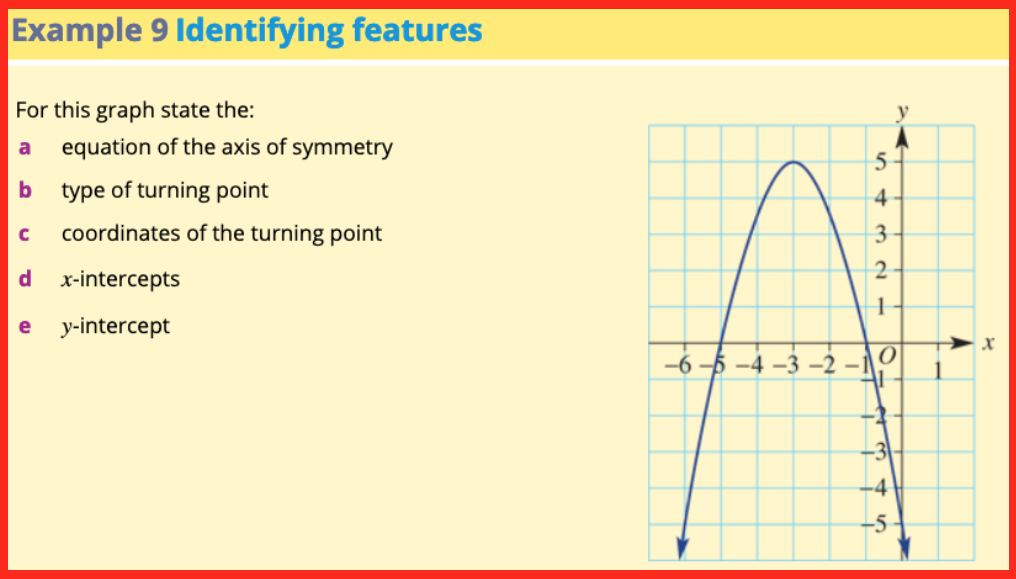## Update

The problem, as commenters have indicated below, is that there is no parabola with the indicated turning point and intercepts. Normally, we’d write this off as a funny but meaningless error. But, coming at the very beginning of the introduction to the parabola, it most definitely qualifies as crap.

## WitCH 13: Here for the Ratio

The WitCH below is courtesy of a clever Year 11 student. It is a worked example from Jacaranda’s Maths Quest 11 Specialist Mathematics (2019):Update (11/08/19)

It is ironic that a solution with an entire column of “Think” instructions exhibits so little thought. Who, for example, thinks to “redraw” a diagram by leaving out a critical line, and by making an angle x/2 appear larger than the original x? And it’s downhill from there.

The solution is painfully long, the consequence of an ill-chosen triangle, requiring the preliminary calculation of a non-obvious distance. As Damo indicates, the angle x is easily determined, as in the following diagram: we have tan(x/2) = 1/12, and we’re all but done.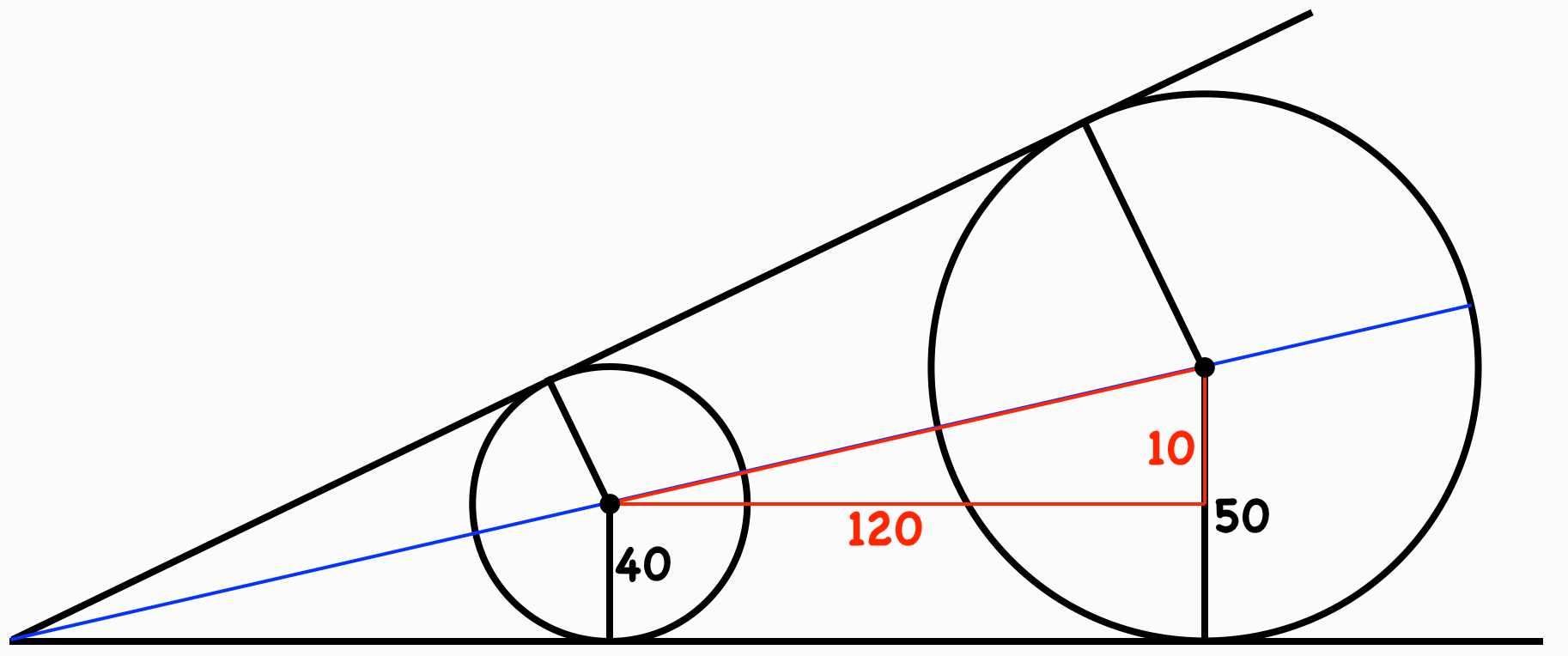(It is not completely obvious that the line through the circle centres makes an angle x/2 with the horizontal, though this follows easily enough from our diagram. The textbook solution, however, contains nothing explicit or implicit to indicate why the angle should be so.)

But there is something more seriously wrong here than the poor illustration of a poorly chosen solution. Consider, for example, Step 5 (!) where, finally, we have a suitable SOHCAHTOA triangle to calculate x/2, and thus x. This simple computation is written out in six tedious lines.

The whole painful six-step solution is written in this unreadable we-think-you’re-an-idiot style. Who does this? Who expects anybody to do this? Who thinks writing out a solution in such excruciating micro-detail helps anyone? Who ever reads it? There is probably no better way to make students hate (what they think is) mathematics than to present it as unforgiving, soulless bookkeeping.

And, finally, as Damo notes, there’s the gratuitous decimals. This poison is endemic in school mathematics, but here it has an extra special anti-charm. When teaching ratios don’t you “think”, maybe, it’s preferable to use ratios?

## PoSWW 4: Overly Complex

This PoSWW comes courtesy of a smart Year 11 VCE student who, it appears, may be a rich source of such nonsense. It’s an exercise in the Jacaranda text MathsQuest 11, Specialist Mathematics (2019).

To be honest, we’re not sure the exercise below is a PoSWW. It may simply be a minor error, the likes of which are inevitable in any text, and of which it is uninteresting and unfair to nitpick. But, for the life of us, we have no idea what the authors might have intended to ask. Make of it what you will:UPDATE: For those hoping that context will help make sense of the exercise, the section of the text is an introduction to factoring over complex numbers. And, the text’s answer to the above exercise is A = 2, B = 5, C = -1, D = 2.

## WitCH 9: A Distant Hope

This WitCH (as is the accompanying PoSWW) is an exercise and solution from Cambridge’s Mathematical Methods Units 1 and 2, and is courtesy of the Evil Mathologer. (A reminder that WitCH 2, WitCH3, Witch 7 and WitCH 8 are still open for business.)## Update

As Number 8 and Potii pointed out, notation of the form AB is amtriguous, referring in turn to the line through A and B, the segment from A to B and the distance from A to B. (This lazy lack of definition appears to be systemic in the textbook.) And, as Potii pointed out, there’s nothing stopping A being the same point as C.

And, the typesetting sucks.

And, “therefore” dots suck.

## PoSWW 3: Not the Right Angle

This PoSWW (as is the accompanying WitCH) is from Cambridge’s Mathematical Methods Units 1 and 2. and is courtesy of the Evil Mathologer. (A reminder, we continue to post on Cambridge not because their texts are worse than others, but simply because their badness is what we get to see. We welcome all emails with any suggestions for PoSWWs or WitCHes.)

We will update this PoSWW, below, after people have had a chance to comment.## Update

Similar to Witch 6, the above proof is self-indulgent crap, and obviously so. It is obviously not intended to be read by anyone.

One can argue how much detail should be given in such a proof, particularly in a subject and for a curriculum that systemically trashes the concept of proof. But it is difficult to see why the diagram below, coupled with the obvious equations and an easy word, wouldn’t suffice.## WitCH 8: Oblique Reasoning

A reminder, WitCH 2, WitCH 3 and WitCH 7 are also open for business. Our new WitCH comes courtesy of John the Merciless. Once again, it is from Cambridge’s text Specialist Mathematics VCE Units 3 & 4 (2019). The text provides a general definition and some instruction, followed by a number of examples, one of which we have included below. Have fun.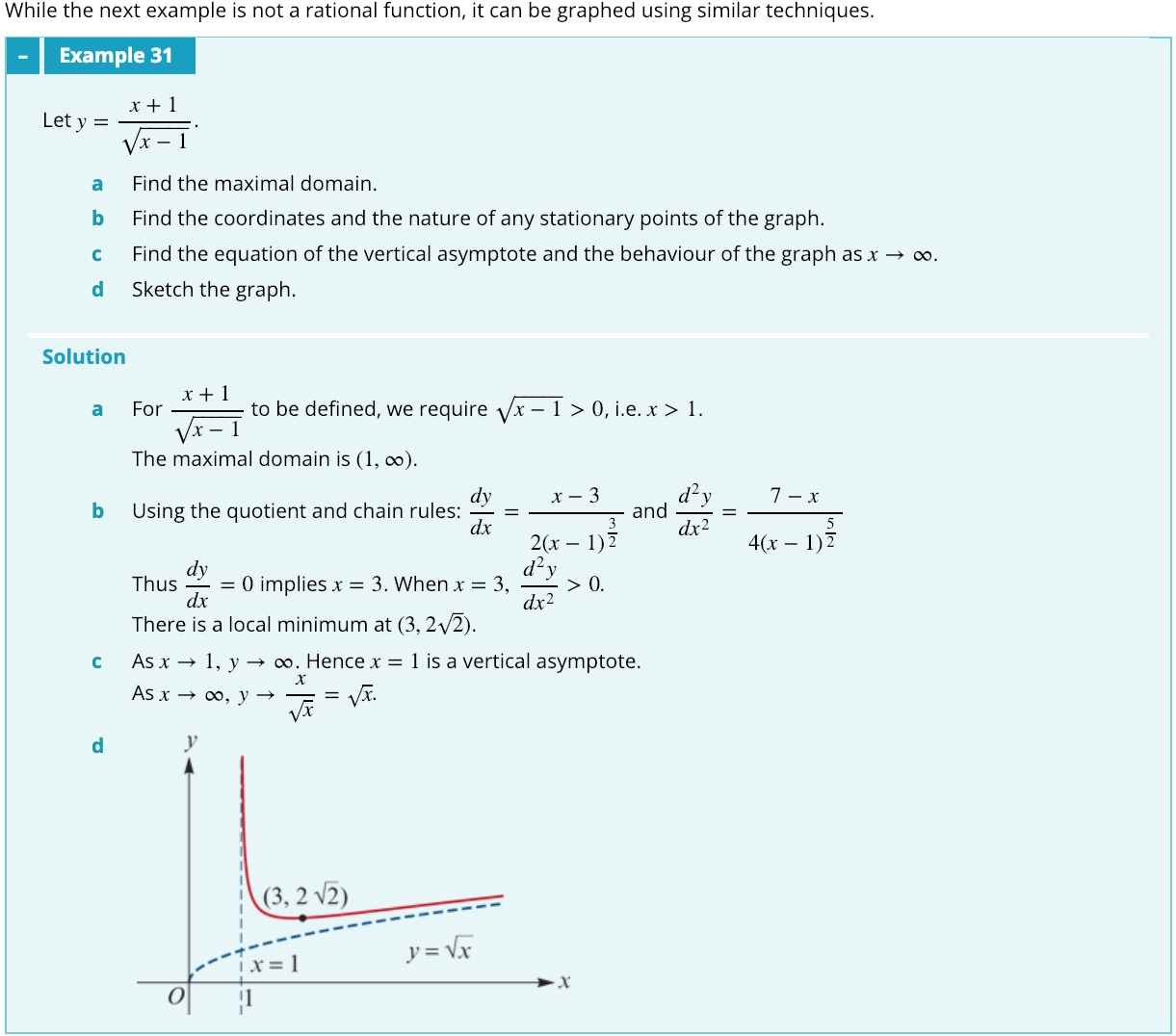Update

With John the Impatient’s permission, I’ve removed John’s comments for now, to create a clean slate. It’s up for other readers to do the work here, and (the royal) we are prepared to wait (as is the continuing case for WitCh 2 and Witch 3).

This WitCH is probably difficult for a Specialist teacher (and much more so for other teachers). But it is also important: the instruction and the example, and the subsequent exercises, are deeply flawed. (If anybody can confirm that  exercise 6G 17(f) exists in a current electronic or hard copy version, please indicate so in the comments.)

Update (05/02/20)

It is obviously long, long past time to sort out this godawful mess. We apologise to all those industrious commenters, who nailed the essential wrongness, and whose hard work was left hanging. This will also be a very long update; you should pour yourself a stiff drink, grab the bottle and get comfy. For the benefit of the Twitter addicts, however, who now find it difficult to concentrate for more than two paragraphs, here’s the punchline:

Cambridge‘s notion of “non-vertical asymptote” is so vague, falling so short of a proper definition, it is close to meaningless and it is pointless. This leads to the claimin Example 31 being flawed in three distinct ways. In particular, it follows from Cambridge that the “curve”is a “non-vertical asymptote” to the function.

The source of Cambridge‘s confusion is also easy to state:

More complicated asymptotes cannot be defined, interpreted or computed in the manner possible for simpler asymptotes.

Unfortunately, the discussion in Cambridge is so sparse and so far from coherent that directly critiquing the excerpt above would probably be incomprehensible. So, we’ll first try to make very careful sense of “non-vertical asymptotes”, taking some minor whacks at Cambridge along the way. Then, with that sense as foundation, it will be easy work to hammer the excerpt above. To simplify the discussion, we’ll only consider the asymptotes of a functionas. Obviously, the case wherecan be handled in like manner, and vertical asymptotes are pretty straightforward.

OK, take a swig and let’s go.

1. Horizontal Asymptotes

We’ll begin with the familiar and demon-free case, for asymptotes as horizontal lines. Consider, for example, the functionWe can write, noting the second term is tiny whenhuge. So, we declare thathas the horizontal asymptote.

Formally, the asymptote in this example is captured with limits. The underlying functional behaviour isor, more officially,. The limit formalism is of no benefit here, however, and is merely likely to confuse. The informal manner of thinking about and writing limits, as is standard in schools, suffices for the understanding of and computation of horizontal asymptotes.

Note also that horizontal asymptotes can easily be spotted with essentially no calculation. Consider the functionwhich is Example 29 in Section 6G of Cambridge. Here, it is only the highest powers, theterms in the numerator and denominator, that matter; thes cancel, and so the function has horizontal asymptote. This simplification also applies to suitable rooty (algebraic) functions. The functionfor example, also has horizontal asymptote, for the same reason.

2. Linear Asymptotes

We’ll now consider general linear asymptotes, which are still reasonably straight-forward (and which include horizontal asymptotes as a special case). There are, however, two demons lurking, one conceptual and one computational.

Cambridge begins with the exampleThe function can be rewritten as, and it seems simple enough: a similar “whenis huge, the leftovers are tiny” intuition leads us to declare thathas the linear asymptote. The trouble is that the example, and the discussion that follows, are too simple, so that the demons remain hidden.

3. A Conceptual Demon

How do we formally (or semi-formally) express that a function has a linear asymptote? For the example above, Cambridge writes “f(x) will approach the line y = 8x – 3”. This is natural, intuitive and sufficiently clear. It is considering the asymptote to be a geometric object, as the line, which is fine at the school level.

What, however, if we want to think of the asymptote as the function? The problem is that bothandare zooming off to infinity, which means that writing, or anything similar, is essentially meaningless. Those arrows are shorthand for limits and, fundamentally, the limit of a function must be a number, not another function. (For vertical asymptotes we consider infinity to be an honorary number, which may seem dodgy, but which can be justified.)

The obvious way around this problem is simply to ignore it. As long as we stick to asymptotes of reasonably simple functions – rational functions and carefully chosen others – Cambridge’s intuitive approach is fine. But in the, um, unlikely event that our situation is not so simple, then we have to carefully consider the limiting behaviour of functions. So, we’ll continue.

Suppose that the functionappears to have the linear functionas asymptote. To capture this idea, the simple trick is to consider the difference of the two functions. Ifis huge then this difference should be tiny, and sobeing the asymptote tois captured by the limit statementAgain, none of this is really necessary for capturing linear asymptotes of simple functions. But it is necessary, at least as underlying guidance, if we wish to consider less simple functions and/or more general asymptotes.

WARNING: It may seem as if our boxed definition of linear asymptotewould work just as well fornon-linear, but there is a trap. There is another, third demon to deal with. Before that, however, our second demon.

4. A Computational Demon

It is very import to understand that the simple, “highest powers” trick we indicated above for spotting horizontal asymptotes does not work for general linear asymptotes. Cambridge‘s introductory exampleillustrates the issue, albeit poorly and with no subsequent examples. For a better illustration, we have Exercise 12 in Section 6G, which presents us with the functionHere, it would be invalid to divide theby theand then declare the linear asymptote to be. The problem is thein the denominator has an effect, and we are forced to perform long division or something similar to determine that effect. So,, and then it is clear that the linear asymptote is.

All that is fine, as far as it goes. Cambridge routinely performs long division to correctly determine linear asymptotes (including horizontal asymptotes, for which the simpler highest powers trick would have sufficed). What, however, if the function is not rational? Consider, for example, Example 31 above. Can we safely ignore thein the denominator of the function, as Cambridge has done? And, if so, why?

In order to stay in the linear world for now, we’ll leave Example 31 and instead consider two other examples:So, are we permitted to ignore theand thein the denominators ofand? It turns out that the answers are “Yes” and “No”: the functionhas linear asymptote, buthas linear asymptote.

The behaviour ofandis, of course, anything but obvious. In particular, the “highest powers” and long division tricks are of no assistance here. Moreover, similar difficulties arise with the analysis of non-horizontal asymptotes of pretty much all rooty functions. It is essentially impossible for a Victorian school text to cover non-horizontal asymptotes of non-rational functions. The text must either work very hard, or it must cheat very hard; Cambridge takes the latter approach.

5. A Nonlinear Demon

Almost there. We have non-linear “asymptotes” left to consider, which are fundamentally demonic. This is demonstrated by Cambridge‘s very first example, Example 26, which considers the functionDividing through bygives, after which Cambridge baldly declares “The non-vertical asymptote has equation“. But why, exactly? All we have to go on is Cambridge‘s intuitive approach toabove. Intuitively, the functionapproaches the parabola. That’s fine, and we can formalise it by setting. Then guided by our boxed definition above, we can write, and we seem to have our asymptote.

The problem is that there are a zillion functions that will fit into that blue box. It is also true, for example, that. This means thatitself just as good an asymptote as. So, yes, one can reasonably declare thatis asymptotic to, but we cannot declareto be THE asymptote to.

Of course we want asymptotes to be unique, so what do we do? There are two ways out of this mess, the first solution being to restrict the type of function that we’ll permit to be an asymptote. That’s intrinsically what we did when considering horizontal and linear asymptotes, and it is exactly why the multiple asymptote problem didn’t arise in those contexts: linear functions only had to compete with other linear functions. Now, for general rational functions, we must broaden the notion of asymptote, but it’s critical to not overdo it: we now permit polynomial asymptotes, but nothing more general. Then, every rational function will have a unique polynomial (possibly linear, possibly horizontal) asymptote.

But now, what do we do for non-rational functions? These functions may naturally have rooty asymptotes, as illustrated by Example 31 above. Such functions can also be dealt with, by suitably and carefully generalising “polynomial asymptote”, but it’s all starting to get fussy and annoying. It is making a second solution much more attractive.

That second way out of this mess is to just give up on unique nonlinear “asymptotes”. Then, our blue box becomes a definition of two functions being “asymptotically equivalent”. We don’t get unique asymptotes, but we do get a clear way to think about the asymptotic behaviour of functions. (You can’t always get what you want, But if you try sometimes, you just might find )

One final comment. The computational demon we mentioned above is of course still around to bedevil non-linear asymptotes. This is well illustrated by Exercise 17, which considers the functionAs discussed by commenters below, this exercise used to have a part (f), asking for the vertical asymptote. That part was deleted in later editions, and it is easy to guess why; thein the denominator ofturns out to matter, so that the “highest powers” technique that Cambridge invalidly employs on such examples gives the wrong answer.

6. The Devil in Cambridge‘s detail

Finally. We can now deal with the Cambridge excerpt above. There is no need to write at length here, since everything follows from the discussion above, as indicated. We begin with a clarification of the punchline:

The claimin Example 31 is triply flawed: it is meaningless (conceptual demon); the calculation is invalid (computational demon); and the conclusion is wrong (nonlinear demon).

To be fair, it turns out that the -1 in the denominator of the function in Example 31 can be ignored, but not because of the highest powers trick that Cambridge appears to employ. This also means y = √x will be the non-linear asymptote to y = (x + 1)/√(x-1) if we suitably generalise the notion of “polynomial asymptote”.

That’s plenty wrong, of course. But to round off, here are the other problems with the excerpt:

• The “graph of y = f(x)” doesn’t approach anything. It is the function that approaches the asymptote.
• Underlying the punchline, the definition of “non-vertical asymptote” is hopelessly vague and does not remotely mean what Cambridge thinks it means.
• We do not “require √(x – 1) > 0; we require x – 1 > 0.

And, we’re done. Thank Christ.

## PoSWW 2: Take a Hike

This Proof of Stupidity Without Words comes courtesy of a smart Year 11 VCE student. It’s an exercise in the Jacaranda text Maths Quest 11 Mathematical Methods (2019). (Just to clarify, the stupidity obviously contains plenty of words; it’s the proof of stupidity that requires no words.)## WitCH 6: Parallel Reality

In this WitCH we will again pick on the Cambridge text Specialist Mathematics VCE Units 3 & 4 (2019): see the extract below. (We’d welcome any email or comment with suggestions of other generally WitCHful texts and/or specific WitCHes.) And, a reminder that there is still plenty left to discover in WitCH 2 , WitCH 3 and Tweel’s Mathematical Puzzle.

Have fun.

Update

Below, we go through the passage line by line, but that fails to capture the passage’s intrinsic awfulness. The passage is, as John put it pithily below, a total fatberg. The passage is worse than wrong; it is clumsy, pompous, circuitous, barely comprehensible and utterly pointless.

Why do this? Why write like this? Sure, ideas, particularly mathematical ideas, can be tricky and difficult to convey; dependence/independence isn’t particularly easy to explain. And sure, we all write less clearly than we might wish on occasion. But, if you write/proofread/edit something that the intended “readers” will obviously struggle to understand, then all you’re doing is either showing off or engaging in a meaningless ritual.

An underlying problem is that the entire VCE topic is pointless. Yes, this is the fault of the idiotic VCAA, not the text, but it has to be said, if only as a partial defence of the text. No purpose is served by including in the curriculum a subtle definition that is not then reinforced in some meaningful manner. Consequently, it’s close to impossible to cover this aspect of the curriculum in an efficient, clear and motivated manner. The text could have been one hell of a lot better, but it probably never could have been good.

OK, to the details. Grab a whisky and let’s go.

• First, a clarification. The definition of “parallel vectors” appears in a slightly earlier part of the text. We included it because it is clearly relevant to the main excerpt. We didn’t intend, however, to suggest that the discussion of dependence began with the “parallel” definition.
• For the given definition of “parallel vectors” it is redundant and distracting to specify that the scalar k not be 0.
• As discussed by Number 8, the definition of “parallel vectors” should not exclude the zero vector. The exclusion may be natural in the context of geometric proofs, but here it is a needless source of fussiness, distraction and error.  As an example of a blatant error, immediately following the above passage the text begins a proposition with “Let a and b be two linearly independent (i.e. not parallel) vectors.” A second and entirely predictable error occurs when the text later goes on to “resolve” an arbitrary vector a into components “parallel” and “perpendicular” to a second vector b.
• The definition of “linear combination” involves a clumsy and needless use of subscripts. Thankfully, though weirdly, subscripts aren’t used in the subsequent discussion. The letters used for the vector variables are changed, however, which is the kind of minor but needless, own-goal distraction that shouldn’t occur.
• No concrete example of linear combination is provided. (The more abstract the ideas, the more critical it is that they be anchored immediately with very specific illustration.)
• It is a bad choice to begin with “linear combination”. That idea is difficult enough, but it also leads to a poor and difficult definition of linear dependence, an unswallowable mouthful: “… at least one of its members [elements? vectors?] can be expressed as a linear combination of [the] other vectors [members? elements?] …” Ugh! What really kills this sentence is the “at least one”which stems from the asymmetry hiccup in the definition. (The hiccup is illustrated, for example, by the three vectors a = 3 + 2j + k, b = 9i + 6j + 3k, c = 2i + 4j + 3k. These vectors are dependent, since b = 3a + 0c is a combination of a and c. Note, however, that c cannot be written as a combination of a and b.)
• As was appropriately done for “linear combination”, the definition of linear dependence should be framed in terms of two or three vectors staring at the reader, not for “a set of vectors”.
• The language of sets is obscure and unnecessary.
• No concrete example of linear dependence is provided. There is not even the specialisation to the case of two and/or three vectors (which, again, is how they should have begun).
• If you’re going to begin with “linear combination” then don’t. But, if you are, then the definition of linear independence should precede linear dependence, since linear independence doesn’t have the asymmetry hiccup: no vector can be written as a combination of the other vectors. Then, “dependent” is defined as not independent.
• No concrete example of linear independence is provided.
• The properly symmetric “examples” are the much preferred definition(s) of dependence.
• The “For example” is weird. It is more accurate to label what follows as special cases. They are not just special cases, however, since they also incorporate non-obvious reworking of the definition of dependence.
• No proof or discussion is provided that the “example[s]”  are equivalent to the definition.
• No genuine example is provided to illustrate the “example[s]”.
• The simple identification of two vectors being parallel/non-parallel if and only if they are dependent/independent is destroyed by the exclusion of the zero vector.
• There is no indication why any set of vectors including the zero vector must be dependent.
• The expression “two-dimensional vector” is lazy and wrong: spaces have dimension, not vectors. (Ditto “three-dimensional vectors”.)
• No proof or discussion is provided that any set of three “two dimensional vectors” is dependent. (Ditto “for three-dimensional vectors”.)
• The “method” for checking the dependence of three vectors is close to unreadable. They could have begun “Let a and b be linearly independent vectors”. (Or, with the correct definition, “Let a and b be non-parallel vectors”.)
• There is no indication of or clarification of or illustration of the subtle distinction between the original “definition” of linear dependence and the subsequent “method”.

What a TARDIS of bullshit.